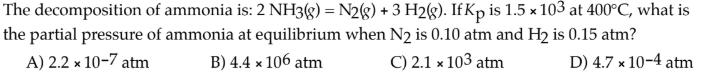# The decomposition of ammonia is: 2 NH3(g) = N2(g) + 3 H2(g). If Kp is 1.5 × 10^3 at 400°C, what is the partial pressure of ammonia at equilibrium when N2 is 0.10 atm and H2 is 0.15 atm? A) 2.2 × 10^-7 atm B) 4.4 × 10^6 atm C) 2.1 × 10^3 atm D) 4.7 × 10^-4 atm